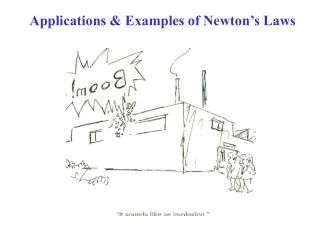DownloadDownload PresentationApplications & Examples of Newton’s Laws

# Applications & Examples of Newton’s Laws

Download Presentation## Applications & Examples of Newton’s Laws

- - - - - - - - - - - - - - - - - - - - - - - - - - - E N D - - - - - - - - - - - - - - - - - - - - - - - - - - -
##### Presentation Transcript

1. Applications & Examples of Newton’s Laws

2. Forces are VECTORS!! • Newton’s 2nd Law: ∑F = ma ∑F = VECTORSUMof all forces on mass m  Need VECTORaddition to add forces in the 2nd Law! • Forces add according to rules of VECTOR ADDITION! (Ch. 3)

3. Newton’s 2nd Law problems: • STEP 1:Sketch the situation!! • Draw a “Free Body” diagram for EACHbody in problem & draw ALL forces acting on it. • Part of your grade on exam & quiz problems! • STEP 2:Resolve the forces on each body into components • Use a convenient choice of x,y axes • Use the rules for finding vector components from Ch. 3.

4. STEP 3: Apply Newton’s 2nd Law to EACH BODY SEPARATELY: ∑F = ma • A SEPARATE equation like this for each body! • Resolved into components: ∑Fx = max ∑Fy = may Notice that this is theLASTstep, NOTthe first!

5. Conceptual Example Moving at constant v, withNO friction, which free body diagram is correct?

6. ExampleParticle in Equilibrium “Equilibrium” ≡The total force is zero. ∑F = 0 or ∑Fx = 0 & ∑Fy = 0 Example (a) Hanging lamp (massless chain). (b) Free body diagram for lamp. ∑Fy = 0  T – Fg = 0; T = Fg = mg (c) Free body diagram for chain. ∑Fy = 0  T – T´ = 0; T´ = T = mg

7. ExampleParticle Under a Net Force Example (a) Crate being pulled to right across a floor. (b) Free body diagram for crate. ∑Fx = T = max ax= (T/m) ay= 0, because of no vertical motion. ∑Fy = 0  n – Fg = 0; n = Fg = mg

8. ExampleNormal Force Again “Normal Force” ≡When a mass is in contact with a surface, the Normal Force n = force perpendicular to (normal to) the surface acting on the mass. Example Book on a table. Hand pushing down. Book free body diagram.  ay= 0, because of no vertical motion (equilibrium). ∑Fy = 0  n – Fg - F = 0  n = Fg + F = mg + F Showing again that the normal force is not always = & opposite to the weight!!

9. Example 5.4: Traffic Light at Equilibrium (a) Traffic Light, Fg = mg = 122 N hangs from a cable, fastened to a support. Upper cables are weaker than vertical one. Will break if tension exceeds 100 N. Does light fall or stay hanging? (b) Free body diagram for light. ay= 0, no vertical motion. ∑Fy = 0  T3 – Fg = 0 T3 = Fg = mg = 122 N (c) Free body diagram for cable junction (zero mass).T1x = -T1cos(37°), T1y = T1sin(37°) T2x = T2cos(53°), T2y = T2sin(53°), ax= ay= 0. Unknowns are T1 & T2. ∑Fx = 0  T1x + T2x = 0 or -T1cos(37°) + T2cos(53°) = 0 (1) ∑Fy = 0  T1y + T2y – T3 = 0 orT1sin(37°) + T2sin(53°) – 122 N = 0 (2) (1) & (2) are 2 equations, 2 unknowns.Algebrais required to solve for T1& T2! Solution: T1 = 73.4 N, T2 = 97.4 N

10. Example

11. Example 5.6: Runaway Car

12. Example 5.7: One Block Pushes Another

13. Example 5.8: Weighing a Fish in an Elevator

14. Example 5.9: Atwood Machine

15. Example 5.10Inclined Plane, 2 Connected Objects